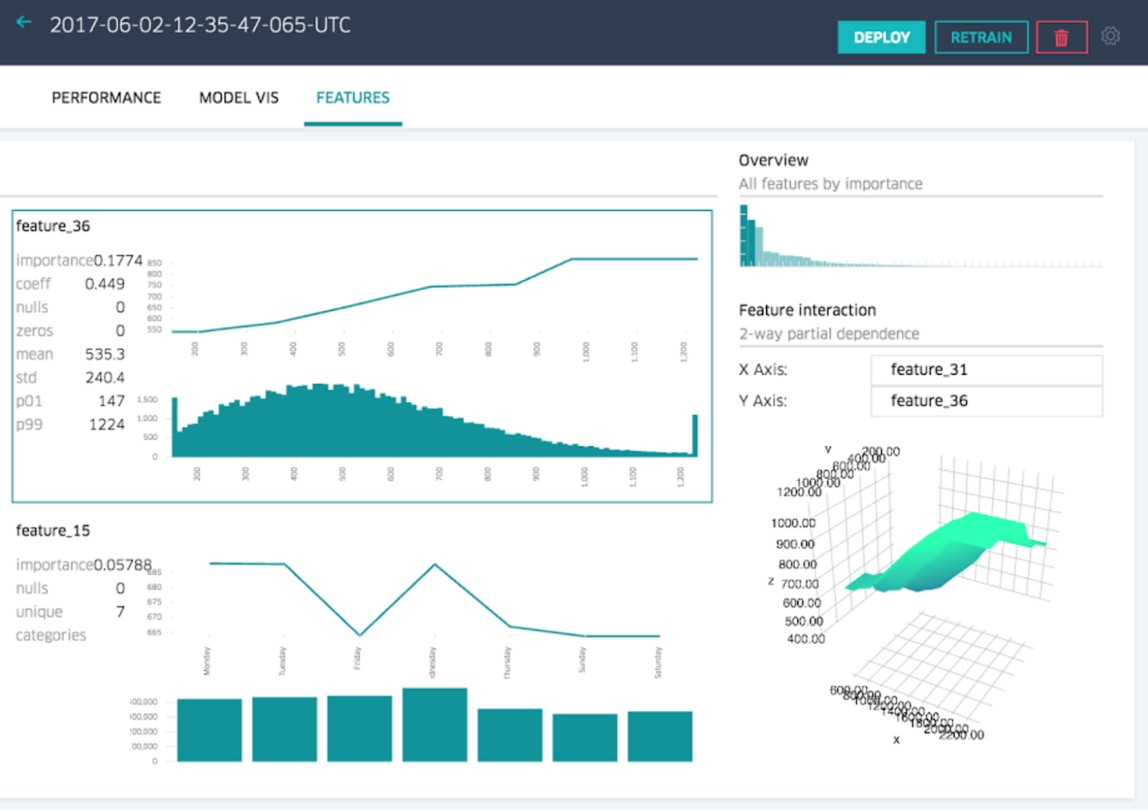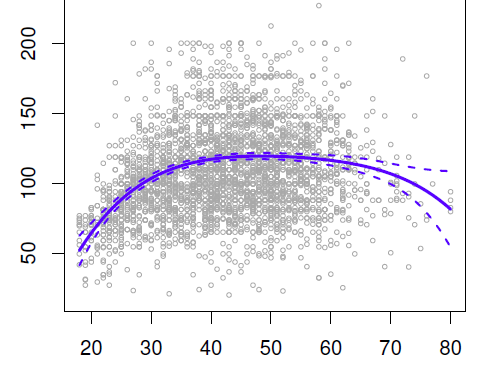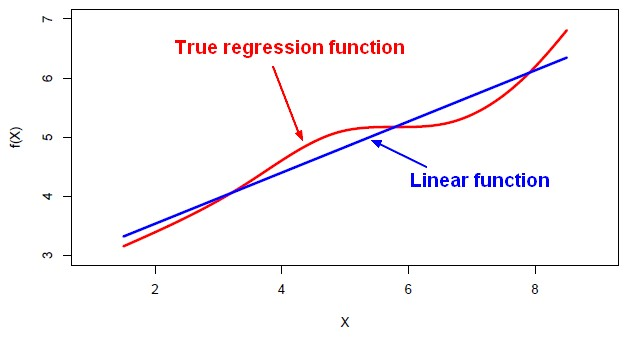# Statistics - (Regression Coefficient|Weight|Slope) (B)

The regression coefficients, the slopes, or, the B values represent the unique variants explained in the outcome by each predictor.

The regression coefficient, $B_1$ is the slope for $X_1$ . assuming an average score on any other predictor $X_2, X_3, \dots, X_n$ . If there's no moderation, it's representative of all the other values on all the other predictors. Like the mean is representative of the entire sample, if you don't have any skew or outliers effects.

No moderation effect implies that $B_1$ is consistent across the entire distribution of others predictors.

Having a moderation effect, implies that a single regression coefficient relating x to y is not sufficient. Because the slope representing X to Y is actually changing as a function of this moderator variable, Z. So one regression coefficient B1 is not sufficient to count for the true relationship that exists between X and Y. A moderator variable is needed to show that the relationship is changing as a function of it. That's the power of a moderation analysis.

While interpreting the slopes, you have to take the units of the variables into account.

Recommended Pages(Mathematics|Statistics) - Statistical Parameter

population parameter A parameter is a numerical characteristic, feature, or measurable factor that help in defining a particular model. Unlike variables, parameters are not listed among the arguments...Convex

Log(loss) is convex, which means that we can use gradient descent to find weights that result in a global minimum. 0 / 1 loss is not convex due to its abrupt decision boundary at z = 0, so it is difficult...Data Mining - (Attribute|Feature) (Selection|Importance)

Feature selection is the second class of dimension reduction methods. They are used to reduce the number of predictors used by a model by selecting the best d predictors among the original p predictors....Data Mining - (Global) Polynomial Regression (Degree)

polynomials regression Although polynomials are easy to think of, splines are much better behaved and more local. With polynomial regression, you create new variables that are just transformations...Data Mining - (Stochastic) Gradient descent (SGD)

Gradient descent can be used to train various kinds of regression and classification models. It's an iterative process and therefore is well suited for map reduce process. The gradient descent update...Linear Algebra - Linear Equation

A linear equation represents a linear function that forms a straight line. A common form of a linear equation in the two variables (two dimensions) x and y is where: m is a constant named the slope...Machine Learning - Linear (Regression|Model)

Linear regression is a regression method (ie mathematical technique for predicting numeric outcome) based on the resolution of linear equation. This is a classical statistical method dating back more...Math - Derivative (Sensitivity to Change, Differentiation)

The derivative of a function of a real variable measures the sensitivity to change of a quantity (a function or dependent variable) which is determined by another quantity (the independent variable). ...R - Multiple Linear Regression

Multiple linear regression with R functions such as lm Unstandardized Multiple Regression Regression analyses, standardized (in the z scale). The point is a short-cut to select all variables....R - Simple Linear Regression

simple linear regression with R function such as lm Unstandardized Simple Regression Regression analyses, standardized (in the z scale). In simple regression, the standardized regression coefficient...